Courses

# Scalars and Vectors Mechanical Engineering Notes | EduRev

## Mechanical Engineering : Scalars and Vectors Mechanical Engineering Notes | EduRev

The document Scalars and Vectors Mechanical Engineering Notes | EduRev is a part of the Mechanical Engineering Course Engineering Mechanics - Notes, Videos, MCQs & PPTs.
All you need of Mechanical Engineering at this link: Mechanical Engineering

Scalars and Vectors

We use two kinds of quantities in mechanics—scalars and vectors. Scalar quantities are those with which only a magnitude is associated. Examples of scalar quantities are time, volume, density, speed, energy, and mass. Vector quantities, on the other hand, possess direction as well as magnitude, and must obey the parallelogram law of addition as described later in this article. Examples of vector quantities are displacement, velocity, acceleration, force, moment, and momentum. Speed is a scalar. It is the magnitude of velocity, which is a vector. Thus velocity is speciﬁed by a direction as well as a speed.

Vectors representing physical quantities can be classiﬁed as free, sliding, or ﬁxed.

A free vector is one whose action is not conﬁned to or associated with a unique line in space. For example, if a body moves without rotation, then the movement or displacement of any point in the body may be taken as a vector. This vector describes equally well the direction and magnitude of the displacement of every point in the body. Thus, we may represent the displacement of such a body by a free vector.

A sliding vector has a unique line of action in space but not a unique point of application. For example, when an external force acts on a rigid body, the force can be applied at any point along its line of action without changing its effect on the body as a whole,* and thus it is a sliding vector.

A ﬁxed vector is one for which a unique point of application is speciﬁed. The action of a force on a deformable or nonrigid body must be speciﬁed by a ﬁxed vector at the point of application of the force. In this instance the forces and deformations within the body depend on the point of application of the force, as well as on its magnitude and line of action.

Rectangular components are convenient for finding the sum or resultant R of two forces which are concurrent. Consider two forces F1 and F2 which are originally concurrent at a point O. Figure 2/7 shows the line of action of F2 shifted from O to the tip of F1 according to the triangle rule of Fig. 2/3. In adding the force vectors F1 and F2, we may write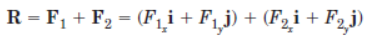or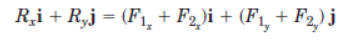from which we conclude that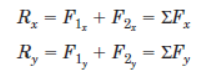(2/4)

The term ΣFx means “the algebraic sum of the x scalar components”.For the example shown in Fig. 2/7, note that the scalar component F2y would be negative.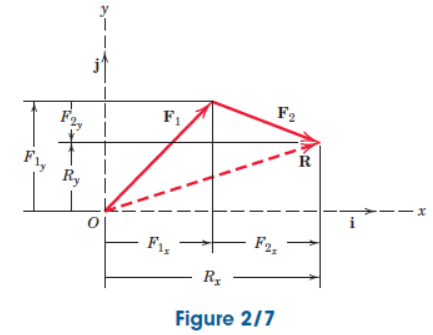Sample Problem 2/1

The forces F1, F2, and F3, all of which act on point A of the bracket, are specified in three different ways. Determine the x and y scalar components of each of the three forces.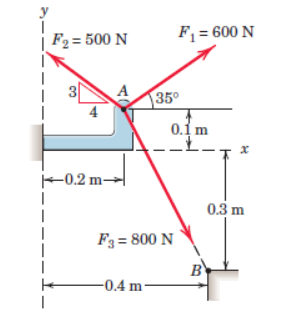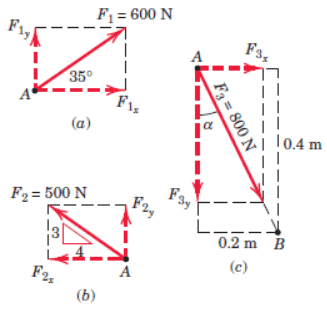Solution. The scalar components of F1, from Fig. a, are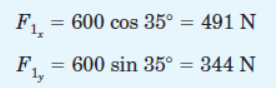Ans

The scalar components of F2, from Fig. b, are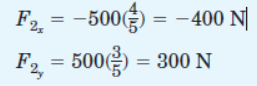Ans

Note that the angle which orients F2 to the x-axis is never calculated. The cosine and sine of the angle are available by inspection of the 3-4-5 triangle. Also note
that the x scalar component of F2 is negative by inspection.

The scalar components of F3 can be obtained by first computing the angle α of Fig. c.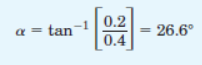Then,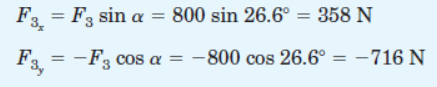Ans

Alternatively, the scalar components of F3 can be obtained by writing F3 as a magnitude times a unit vector nAB in the direction of the line segment AB.
Thus,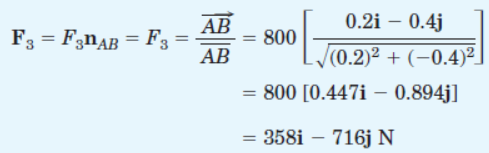The required scalar components are then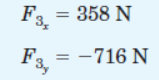Ans.

which agree with our previous results.

Sample Problem 2/2

Combine the two forces P and T, which act on the fixed structure at B, into a single equivalent force R.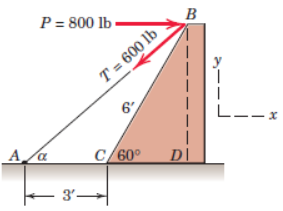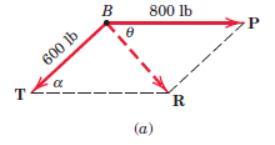Graphical solution. The parallelogram for the vector addition of forces T and P is constructed as shown in Fig. a. The scale used here is 1 in. =800 lb; a scale of 1 in. = 200 lb would be more suitable for regular-size paper and would give greater accuracy. Note that the angle a must be determined prior to construction of the parallelogram. From the given figure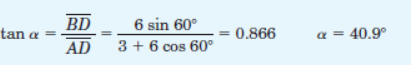Measurement of the length R and direction θ of the resultant force R yields the approximate results

R = 525 lb θ = 49o  Ans.

Geometric solution. The triangle for the vector addition of T and P is shown in Fig. b. The angle α is calculated as above. The law of cosines gives

R2 = (600)2 + (800)2 - 2(600)(800) cos 40.9o= 274,300

R = 524 lb   Ans.

From the law of sines, we may determine the angle θ which orients R. Thus,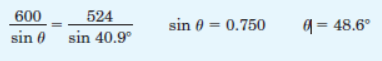Ans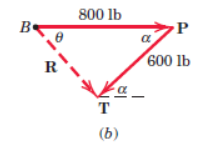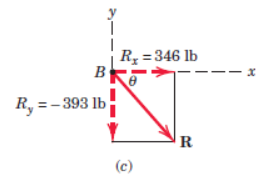Algebraic solution. By using the x-y coordinate system on the given figure, we may write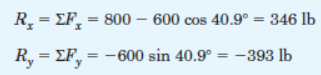The magnitude and direction of the resultant force R as shown in Fig. c are then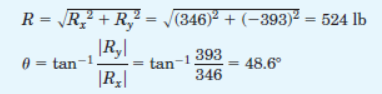Ans.

The resultant R may also be written in vector notation as

R = Rxi + Ryj = 346i - 393j lb  Ans.

Sample Problem 2/3

The 500-N force F is applied to the vertical pole as shown. (1) Write F in terms of the unit vectors i and j and identify both its vector and scalar components. (2) Determine the scalar components of the force vector F along the x'- and y'-axes. (3) Determine the scalar components of F along the x- and y'-axes.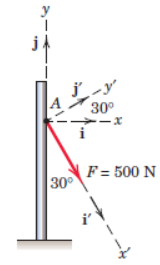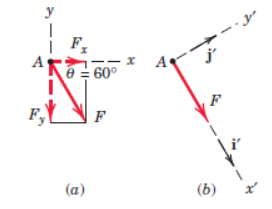Solution. Part (1). From Fig. α we may write F as

F =(F cosθ)i - (F sin θ)j

= (500 cos 60o)i - (500 sin 60o)j

= (250i - 433j) N     Ans.

The scalar components are Fx = 250 N and Fy = -433 N. The vector components are Fx = 250i N and Fy=-433j N.

art (2). From Fig. b we may write F as F = 500i' N, so that the required scalar components are

Fx'= 500 N Fy'= 0

Part (3). The components of F in the x- and y'-directions are nonrectangular and are obtained by completing the parallelogram as shown in Fig. c. The magnitudes of the components may be calculated by the law of sines. Thus,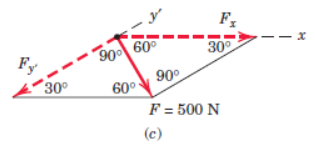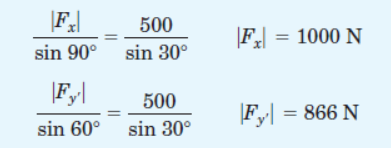The required scalar components are then

Fx = 1000 N Fy'=-866 N         Ans.

Offer running on EduRev: Apply code STAYHOME200 to get INR 200 off on our premium plan EduRev Infinity!

## Engineering Mechanics - Notes, Videos, MCQs & PPTs

30 videos|72 docs|65 tests

,

,

,

,

,

,

,

,

,

,

,

,

,

,

,

,

,

,

,

,

,

;